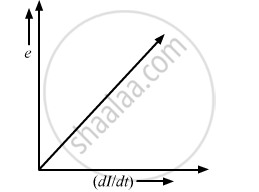# The Current Flowing Through an Inductor of Self Inductance L is Continuously Increasing. Plot a Graph Showing the Variation of Induced Emf Versus di/dt - Physics

The current flowing through an inductor of self-inductance L is continuously increasing. Plot a graph showing the variation of

Induced emf versus dI/dt

#### Solution

Induced emf,

e=(dphi)/dt=-d/dt(LI)

i.e e=-L(dI)/dtConcept: Induced Emf and Current
Is there an error in this question or solution?